Multi-Level MOSFET Driver (Version 8.0+)

The SIMPLIS MOSFET driver has multiple levels for use in different simulation objectives:

• Level 0 : The output switches are modeled using on/off resistances RON1. This is a useful model if the actual switching waveforms are not important, such as for loop gain measurements.
• Level 1 : The output switches are modeled using transistor switches where the current is limited to the parameter I3. For driver currents below the I3 current, the switch is modeled by a resistance equal to the RON1parameter. This model and the level 2 model are used for switching losses.
• Level 2 : The output switches are modeled as 3-segment PWL resistors. The current vs. voltage characteristics can be tailored for different driver implementations using the additional RON2and V1parameters.

In this topic:

Model Name: Multi-Level MOSFET Driver
Simulator: This device is compatible with the SIMPLIS simulator.
Parts Selector Menu Location: MOSFET Drivers > Multi-Level MOSFET Driver (Version 8.0+)
Symbol Library: simplis_analog_functions.sxslb (some symbols are automatically drawn when placed)
Model File: simplis_analog_functions.lb
Subcircuit Name: SIMPLIS_MULTI_LEVEL_DRIVER
Symbols:
 Level 0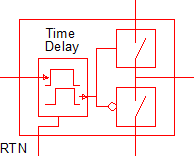Level 1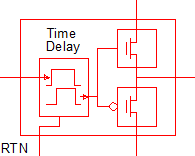Level 2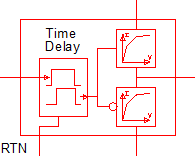Multiple Selections: Multiple devices can be selected and edited simultaneously.

Previous Version Compatibility

This symbol and the electrical model was introduced with version 8.0 and will not simulate in releases prior to Version 8.0.

For a MOSFET driver compatible with versions prior to version 8.0, see the parts selector entry:

Obsolete > MOSFET Drivers > Totem-Pole Output Driver (Obsolete)

The obsolete driver behaves like the Multi-Level MOSFET Driver when the level parameter is set to 0.

Symbol Migration

Symbols placed on schematics in previous versions of SIMetrix/SIMPLIS can be automatically migrated to use the new symbols. The schematic tools menu Tools > Migrate Schematic Symbols to Version 8.20 will invoke a routine which migrates the existing symbols to the new symbols. As this action makes substantial changes to the schematic, it is recommended that you save a backup copy of the schematic first.

Level 0 Driver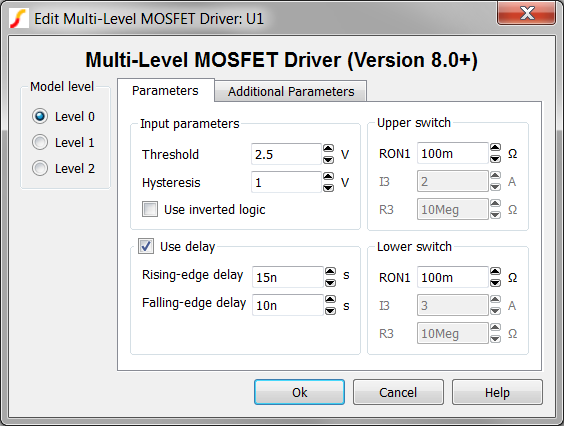Level 0 MOSFET Driver

 Parameters Units Description Input Parameters ThresholdHysteresis V The Threshold, in conjunction with the Hysteresis parameter sets the input voltage thresholds: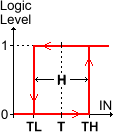TH=T+H/2 TL=T-H/2 where T = Threshold, and H = Hysteresis Use Delay n/a Determines whether or not to use a delay. Rising-edge Delay s The delay from the input rising edge to the initiation of output voltage change. The delay is independent of the logical inversion, and is always applied to the rising edge of the input. Falling-edge Delay s The delay from the input falling edge to the initiation of output voltage change. The delay is independent of the logical inversion and is always applied to the falling edge of the input. Upper switch RON1 Ω The on resistance of the driver transistor. I3 n/a These parameters do not apply to the Level 0 model. R3 n/a Lower switch RON1 Ω The on resistance of the driver transistor. I3 n/a These parameters do not apply to the Level 0 model. R3 n/a

The Level 0 model uses simple switches with on resistance RON1. The output characteristics of one of these switches is shown below with a schematic diagram of the switch configuration.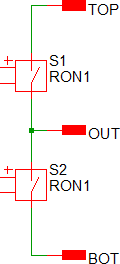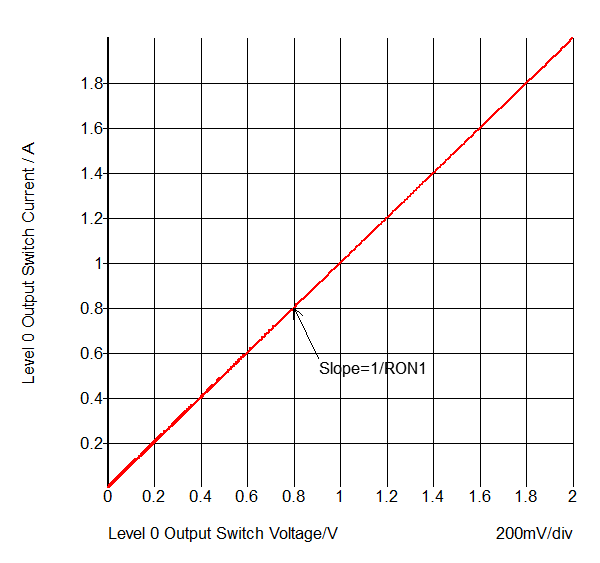Level 1 Driver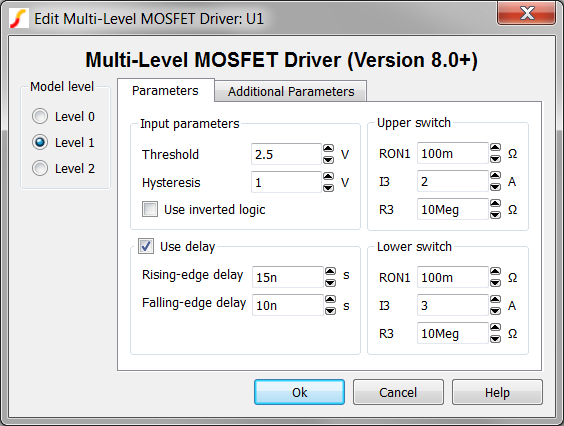Level 1 MOSFET Driver

 Parameters Units Description Input Parameters ThresholdHysteresis V The Threshold, in conjunction with the Hysteresis parameter sets the input voltage thresholds:TH=T+H/2 TL=T-H/2 where T = Threshold, and H = Hysteresis Use Delay n/a Determines whether or not to use a delay. Rising-edge Delay s The delay from the input rising edge to the initiation of output voltage change. The delay is independent of the logical inversion, and is always applied to the rising edge of the input. Falling-edge Delay s The delay from the input falling edge to the initiation of output voltage change. The delay is independent of the logical inversion and is always applied to the falling edge of the input. Upper switch RON1 Ω The on resistance of the driver transistor. I3 A The peak current of the driver. R3 Ω The resistance of the driver transistor when the driver is sourcing the I3current. This resistance is in parallel with the driver transistor. Lower switch RON1 Ω The on resistance of the driver transistor. I3 A The peak current of the driver. R3 Ω The resistance of the driver transistor when the driver is sourcing the I3current. This resistance is in parallel with the driver transistor.

The Level 1 model uses transistor switches which model both on resistance RON1, and peak current I3. When the switch is sourcing/sinking the peak current, a parallel output resistance R3 is present across the switch terminals. The output characteristics of one of these switches is shown below with a schematic diagram of the switch configuration.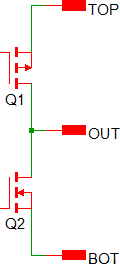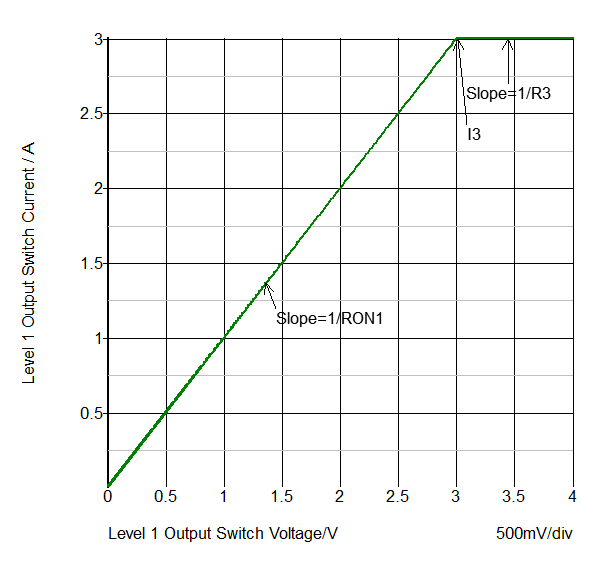Level 2 Driver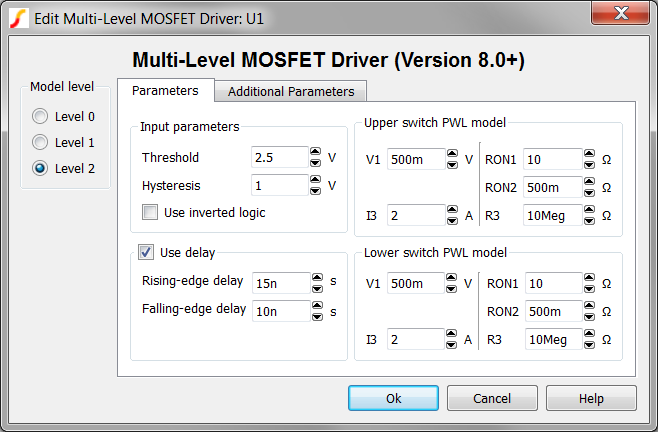Level 2 MOSFET Driver

 Parameters Units Description Input Parameters ThresholdHysteresis V The Threshold, in conjunction with the Hysteresis parameter sets the input voltage thresholds:TH=T+H/2 TL=T-H/2 where T = Threshold, and H = Hysteresis Use Delay n/a Determines whether or not to use a delay. Rising-edge Delay s The delay from the input rising edge to the initiation of output voltage change. The delay is independent of the logical inversion, and is always applied to the rising edge of the input. Falling-edge Delay s The delay from the input falling edge to the initiation of output voltage change. The delay is independent of the logical inversion and is always applied to the falling edge of the input. Upper switch PWL model V1 V The voltage where the driver transitions from the on resistance segment to the RON2 resistance segment. I3 A The peak current of the driver. RON1 Ω The on resistance of the driver transistor. RON2 Ω The resistance of the second segment in the PWL definition. R3 Ω The resistance of the driver transistor when the driver is sourcing the I3 current. Lower switch PWL model V1 V The voltage where the driver transitions from the on resistance segment to the RON2 resistance segment. I3 A The peak current of the driver. RON1 Ω The on resistance of the driver transistor. RON2 Ω The resistance of the second segment in the PWL definition. R3 Ω The resistance of the driver transistor when the driver is sourcing the I3 current.

The Level 2 model uses PWL resistors to model the on resistance with two segments RON1 and RON2, and the peak current I3. When the switch is sourcing the peak current, a parallel output resistance R3 is present across the switch terminals. This model level can be used to model a composite NPN/MOSFET switch configuration as shown below with a schematic diagram below.

Composite NPN/MOSFET Transistor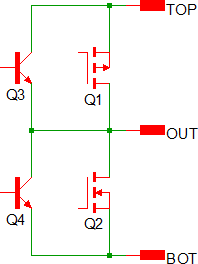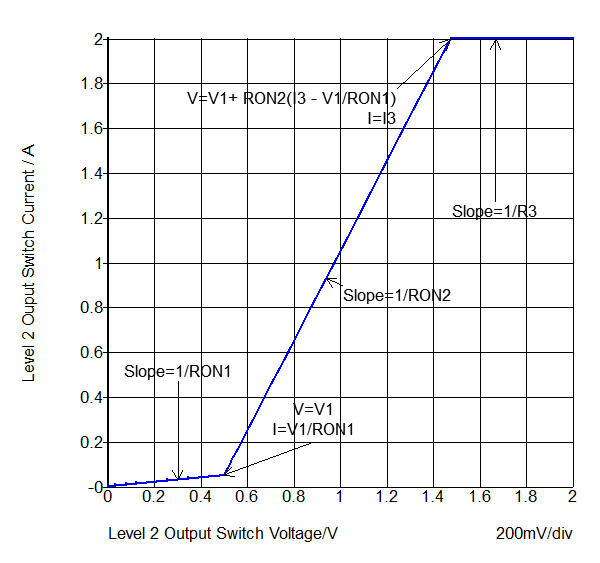Detailed MOSFET Transistor

A more detailed MOSFET transistor can be modeled by changing the RON1 and RON2 parameters. In the below graph, the RON1 parameter is 444mΩ and the RON2 parameter to 1.12Ω.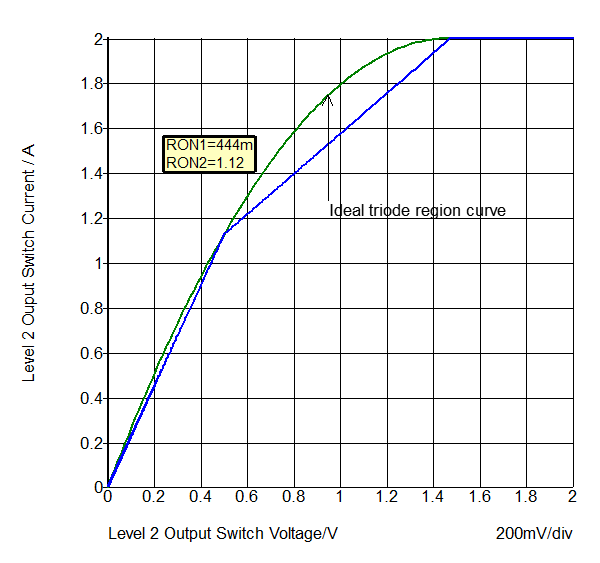Example

The test circuit used to generate the waveform examples in the next section can be downloaded here: simplis_068_multi_level_mosfet_driver.zip. Included in the zip archive is a schematic and the multi-step configuration file. The waveforms in the next section were taken from a multi-step simulation where the level parameter was stepped from 0 to 2, generating three output curves. Other than the level parameter, the driver uses the default values for all parameters. For information on the Multi-Step Analysis see Advanced SIMPLIS Training Course: 3.1 Multi-Step Simulations.

A similar driver is covered in the Advanced SIMPLIS Training Course: 7.0 MOSFET Driver Model.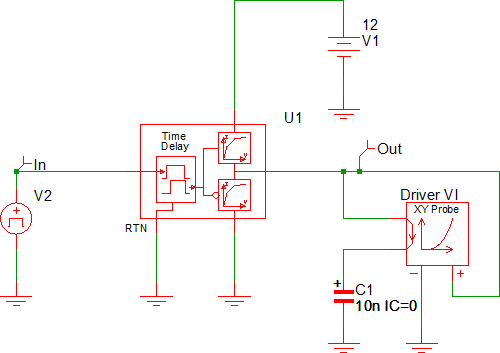Waveforms - Time Domain

The MOSFET driver output waveforms for each of the three model levels is shown below.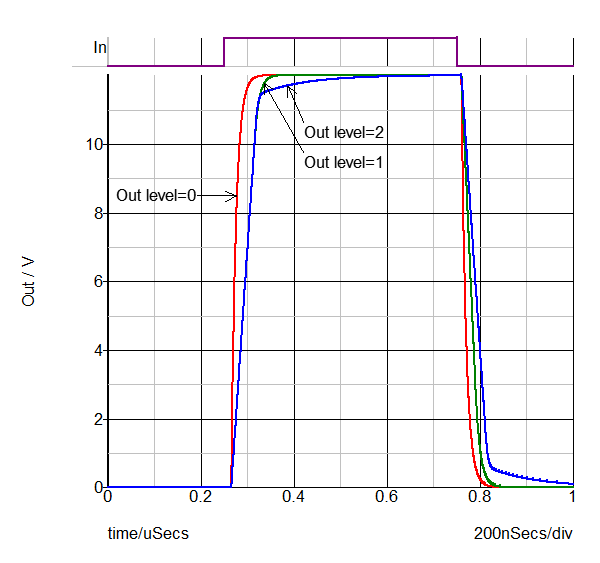Waveforms - XY Output Characteristics

The MOSFET Driver output current is plotted against the output voltage in the graph below. This graph is useful to gain an in-depth understanding how the driver works.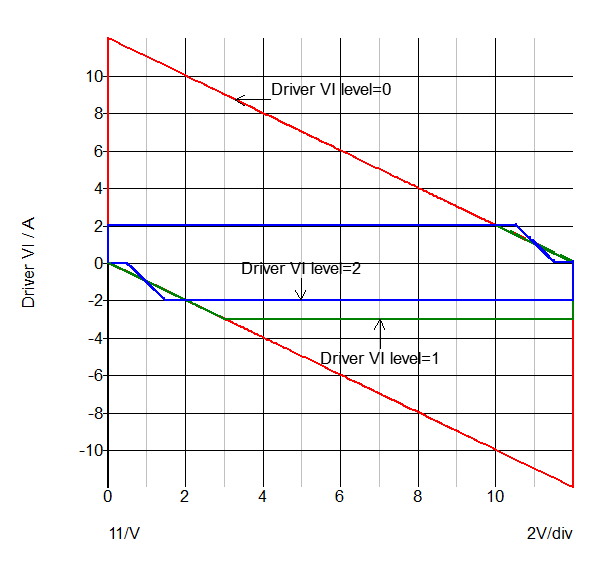Level 0 Operation

Following the red curve which is for the Level 0 model, the driver starts with the output voltage at 0V and driver current at 0A.

• When the input voltage transitions to the high state, the sourcing switch turns on and the driver current is limited only by the resistance of the sourcing switch, RON1. The current spikes to 12A (12V/1Ω).
• As the output voltage rises, the current linearly decreases on a load line of 1Ω until the output voltage reaches 12V.
• When the input voltage transitions to the low state, the process repeats but in the sinking direction, using the sinking RON1.

Level 1 Operation

The green curve is for the Level 1 model.

• As with the Level 0 model, when the input voltage transitions to the high state, the sourcing switch turns on, but in the level 1 model the sourcing current is limited to I3, which is 2A.
• As the output voltage rises, the current is steady at 2A until the output voltage reaches 10V where the switch enters into a resistive state. As with the Level 0 model, the resistance is 1Ω.
• When the input voltage transitions to the low state, the process repeats but in the sinking direction. The sinking I3is 3A, and when the output voltage reaches 3V, the driver output is effectively a 1Ω resistor to ground.

Level 2 Operation

The blue curve is for the Level 2 model.

• As with the Level 1 model, when the input voltage transitions to the high state, the sourcing switch turns on with a current limit of I3, which is 2A.
• As the output voltage rises, the current is steady at 2A until the output voltage reaches a voltage where the switch enters into the RON2resistive state. This voltage is $V1 + RON2 *(I3 - \frac{V1}{RON1})$ volts lower than the positive rail. For the default parameters, the voltage difference between the positive rail and this voltage is 1.475V.
• When the output voltage reaches V1 volts below the positive rail, the sourcing resistance is RON1.
• When the input voltage transitions to the low state, the process repeats but in the sinking direction.